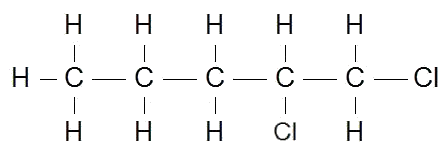CHEM1200

Practice Test 1

Note: These questions are representative of the types you can expect on the test. The actual test will be longer, typically between 20-40 questions.
You will need to bring a SCANTRON and pencil to each class test.

Question 1.Which of the following liquids would make a good solvent for the non-polar substance, iodine, I2?
a. HCl
b. H2O
c. CH3OH
d. NH3
e. pentane

Question 2. Calculate the molality of a solution containing 14.3 g of NaCl in 42.2 g of water
a. 2.45 x 10-4 m
b. 5.80 x 10-4 m
c. 2.45 x 10-1 m
d. 5.79 m
e. 103 m

Question 3. The solubility of CO2 gas in water:
a. increases with increasing temperature
b. decreases with decreasing temperature
c. decreases with increasing temperature
d. is not dependent on temperature

Question 4. Calculate the freezing point of a solution made from 22.0 g of octane (C8H18) dissolved in 148.0.g of benzene. Benzene freezes at 5.50 oC and its Kf value is 5.12 oC/m
a. - 1.16 oC
b. - 0.98 oC
c.   6.66 oC
d.   12.2 oC
e.   5.49 oC

Question 5. A solution that contains 55.0 g ascorbic acid (vitamin C) in 250 g of water freezes at - 2.34 oC. Calculate the molar mass (g/mol) of the solute.
Kf (water) = 1.86 oC/m
a. 1.26
b. 10.9
c. 43.6
d. 175
e. 277

Question 6. Calculate the mole fraction of ethanol (CH3CH2OH, molar mass = 46.0 g/mol) in a solution that contains 46 g of ethanol and 64 g of methanol (CH3OH)
a. 0.33
b. 0.42
c. 0.50
d. 0.67

Question 7. The general formula of an alkane is
a. CnH2n
b. CnH2n+2
c. CnH2n-2
d. CnH2n+4
e. CnH2n-4

Question 8. Which of the following molecules is unsaturated?
a. C3H8
b. CH3
c. C5H10
d. CH4
e. C4H10

Question 9. How many structural isomers are there of C4H10?
a. 2
b. 4
c. 6
d. 8
e. 10

Question 10. The following two molecules are examples of what?

CH3-CH2-O-CH2-CH3      and      CH3-CH2-CH2-CH2-OH
a. isotpes
b. isomers
c. alcohols
d. carboxylic acids
e. unsaturated hydrocarbons

Question 11. Which of the following is the formula for an ether?
a. CH3CHO
b. CH3COCH3
c. CH3OCH3
d. CH3COOH
e. CH2=CH2

Question 12. Which type of organic group does not contain a carbonyl group?
a. ethers
a. carboxylic acids
b. ketones
c. aldehydes
d. esters

Question 13. Which is the most likely main product of the reaction of one mole of HCl wth one mole of CH3-CH2-CH=CH2
a. CH3-CH2-CH=CHCl
b. CH3-CH=CH-CH2Cl
c. CH3-CH2-CH2-CH3
d. CH3-CH2-CH-CH3
|
Cl

Question 14. Which is an organic compound containing the -NH2 group?
a. chloroform
b. citric acid
c. anthracene
d. aniline
e. cresol

Question 15. Which functional group tends to confer acidic properties upon an organic substance?
a. -CHO
b. -CO
c. -CN
d. -COOH
e. -NH2

Question 16. What is the systematic name for the following compound?
Cl
|
CH3-CH2-C-CH3
|
Cl
a. 2-dichlorobutane
b. 2,2-dichlorobutane
c. di-2-chlorobutane
d. di-2,2-dichlorobutane
e. 2-chlorobutane

Question 17. The correct structure for 2,3,3-trimethylpentane isa.
b.
c.
d.

Question 18. The correct name for the following compound isa. 1,1-dichloropentane
b. 1,2-dichloropentane
c. 5,5-dichloropentane
d. 4,5-dichloropentane